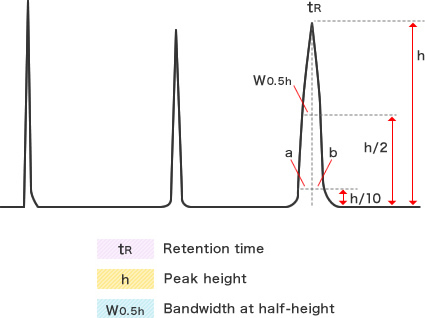Preparative LC systems

# Quality control of self-packed columns

After packing columns, it is recommended that the number of theoretical plates (N), the height equivalent to a theoretical plate (HETP), and the peak asymmetry factor (As) are calculated before use by performing test analyses of standard samples. By regularly and repetitively performing such standard tests, the quality and durability of the packing material can be estimated effectively. If you would like to compare the results between individual tests, you should use the same measuring device under the same conditions in every test. The results could be affected by some factors, such as differences in dead volume, flow rate, temperature, eluent, and sample loading amount.

The formula shown below is available for calculating the number of theoretical plates (N). Larger calculated values of N correspond to higher densities of packing materials in the column. Further, a narrower peak shape at a certain retention time corresponds to a higher N value, and longer column lengths and smaller particle sizes tend to correspond to larger N values. In general, the number of theoretical plates per bed length (m) is often used as an index to estimate the performance of a column.

N　=　5.54 x (tR / W0.5h)2
N/m　＝　N / L L　＝　Column bed length (m)

The height equivalent to a theoretical plate (HETP) is also sometimes used as an index to estimate column performance. The formula shown below is available for calculating HETP. The smaller this value is, the higher the density of packing material packed in the column is.

HETP　＝　L / N

The eluted peak shape is also an important factor for estimating column performance. Determination of the peak asymmetry factor (As) is a relatively simple method for estimating the column performance. The formula shown below is available for calculating As. Values closer to “1” are ideal and indicate higher peak shape symmetry.

As　＝　b / a